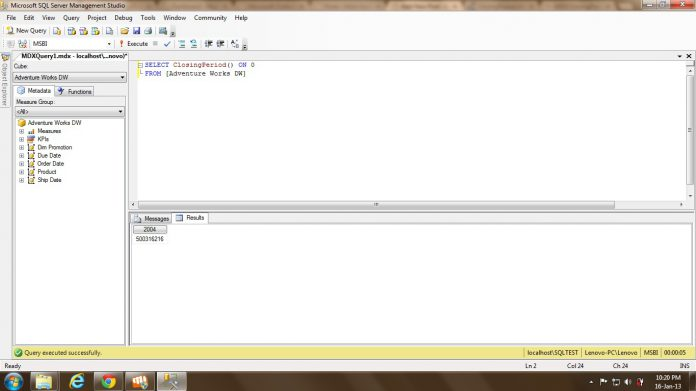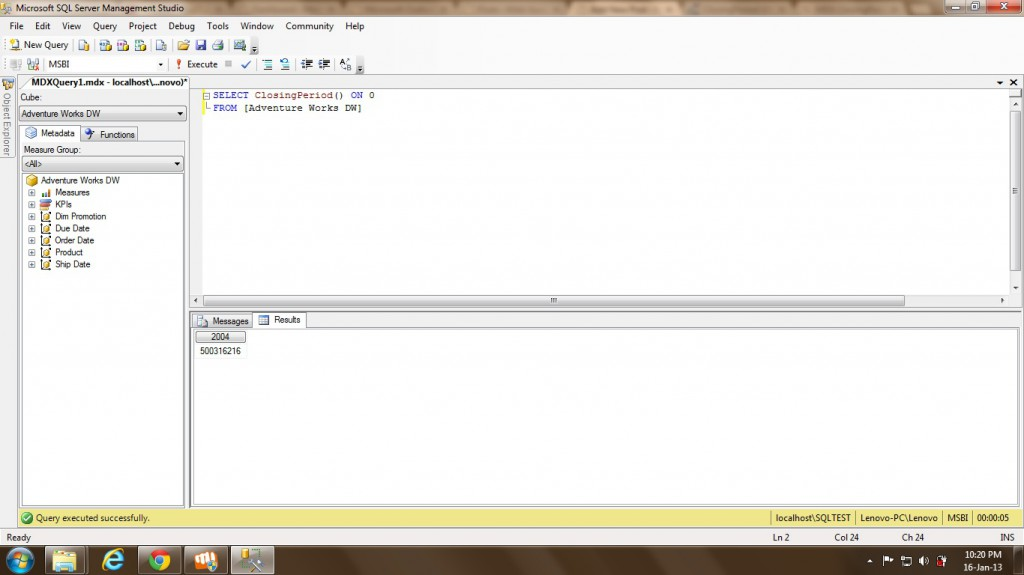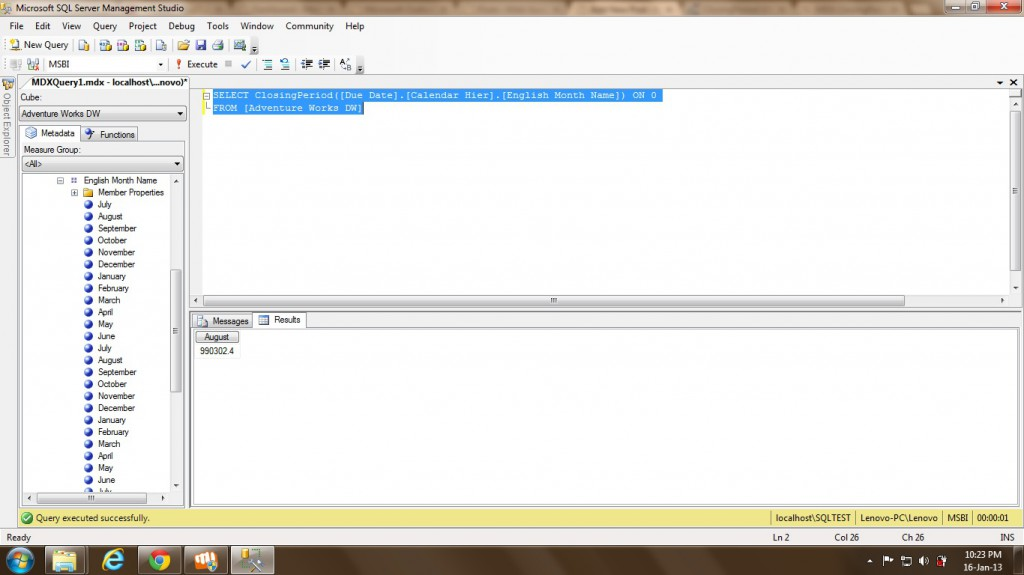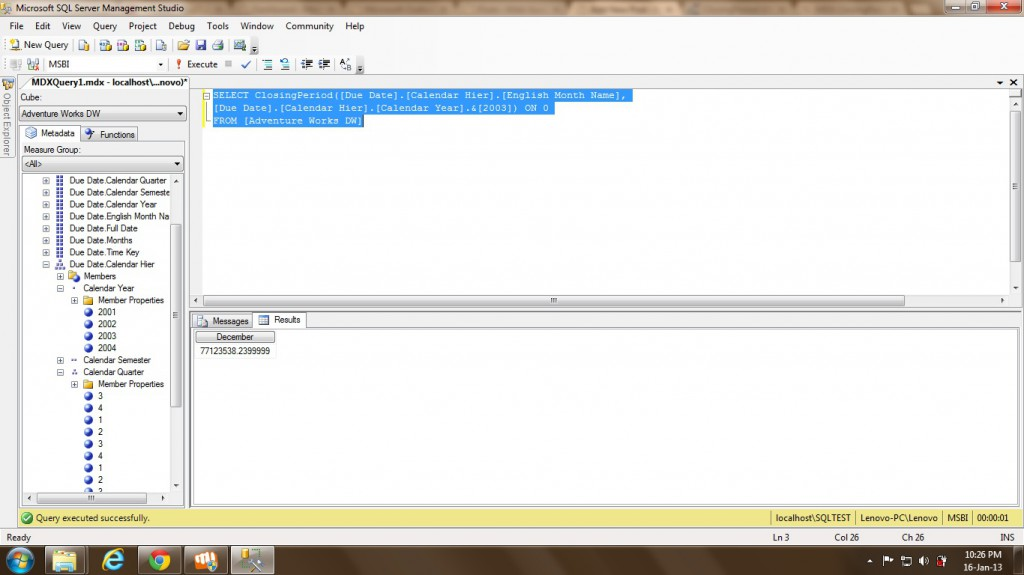# ClosingPeriod function in MDX

5803Friends,

In this post we are gonna discuss about ClosingPeriod function. This is one of the Time functions we have. This function returns the member that is the last sibling among the descendants of a specified member at a specified level.

The syntax is given below –

CLOSINGPERIOD( [«Level»[, «Member»] ] )

Level – A valid MDX expression level.
Member – A valid MDX expression member. This is not a mandatory parameter.

The different possibilities are given below.

• If ONLY level expression is provided then the function returns the last member of the level provided.
• If both level and member is provided then the function returns the last member of the level provided of the supplied Member.
• If none of the parameters are provided then the function returns the last sibling of the default time member if any.

Let’s see the examples of each ..

```SELECT ClosingPeriod() ON 0

When we executed the above query then it returns the default time dimension member last sibling as shown below.When you pass the level to the function as shown below then it returns the last member of the level supplied.

SELECT ClosingPeriod([Due Date].[Calendar Hier].[English Month Name]) ON 0When you pass the level and also member to the function as shown below then it returns the last member of the level supplied for the supplied member.

SELECT ClosingPeriod([Due Date].[Calendar Hier].[English Month Name],
[Due Date].[Calendar Hier].[Calendar Year].&) ON 0